# RD Sharma Solutions for Class 6 Maths Chapter 11: Angles Exercise 11.2

In this exercise, the students mainly gain the idea about the special angles like straight angle, complete angle, zero angle and right angle. It also helps students understand the kinds of angles and methods used to determine them. The solutions prepared by subject experts has step by step explanation in a simple language to make it easier for the students to understand. The students can use RD Sharma Solutions Class 6 Maths Chapter 11 Angles Exercise 11.2 PDF which are provided here to boost their exam preparation.

## RD Sharma Solutions for Class 6 Maths Chapter 11: Angles Exercise 11.2 Download PDF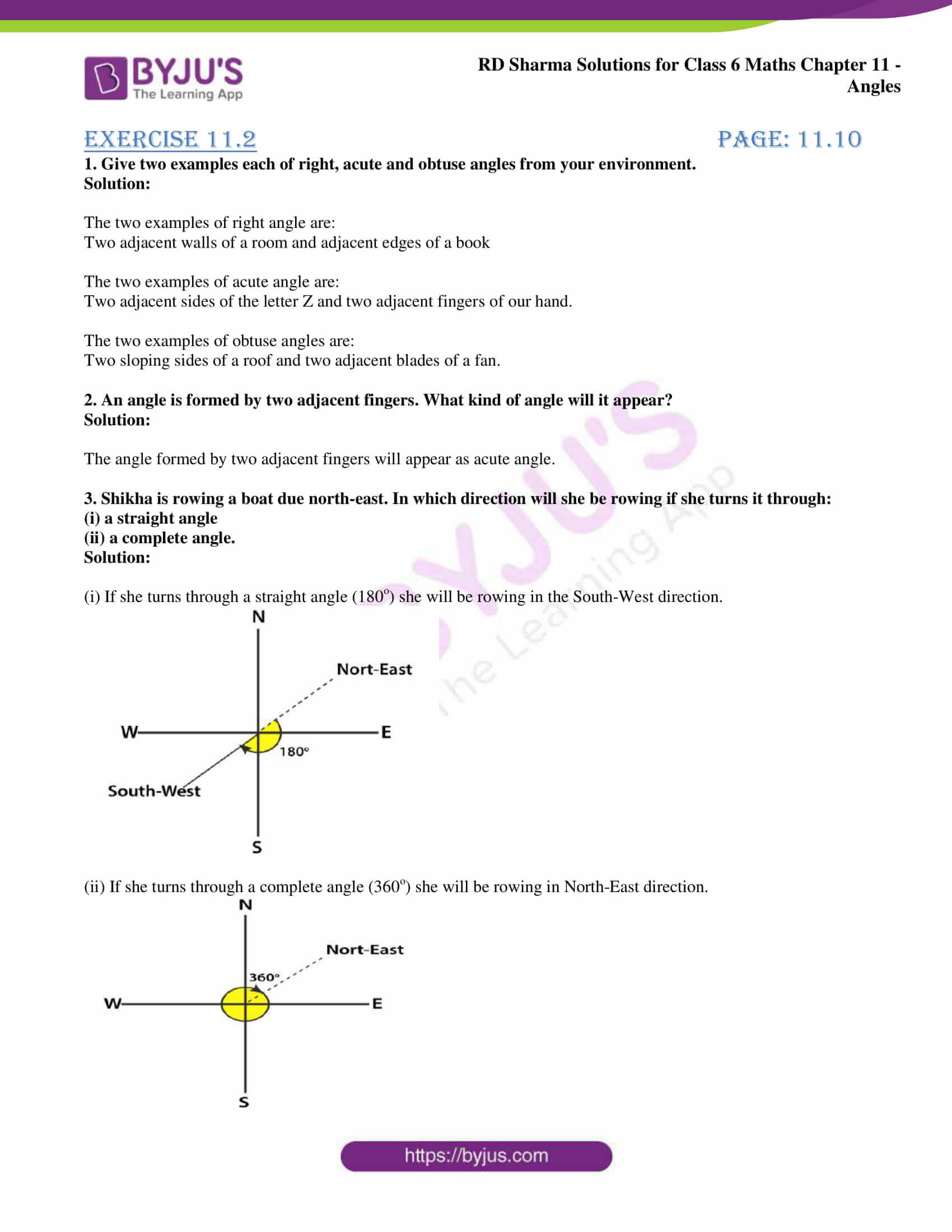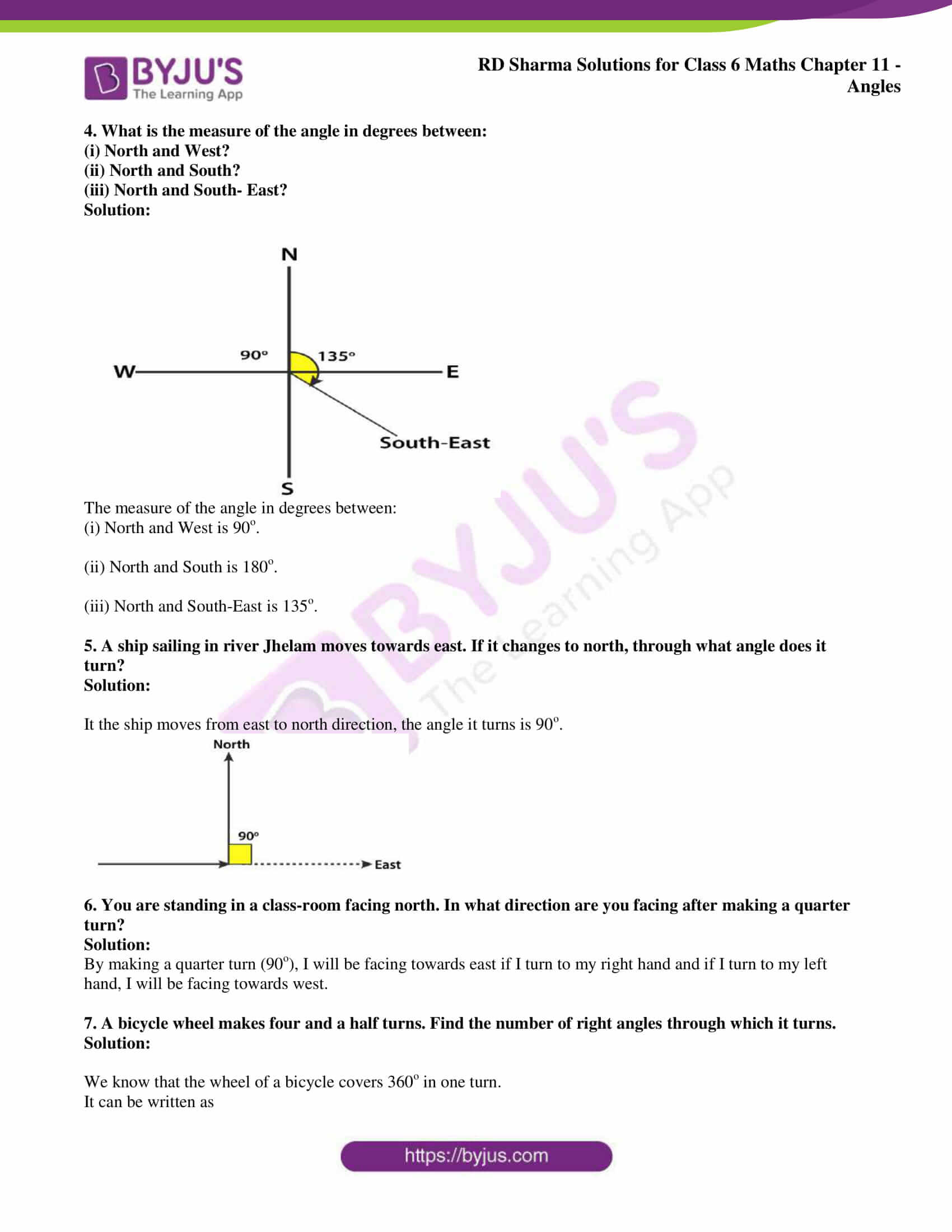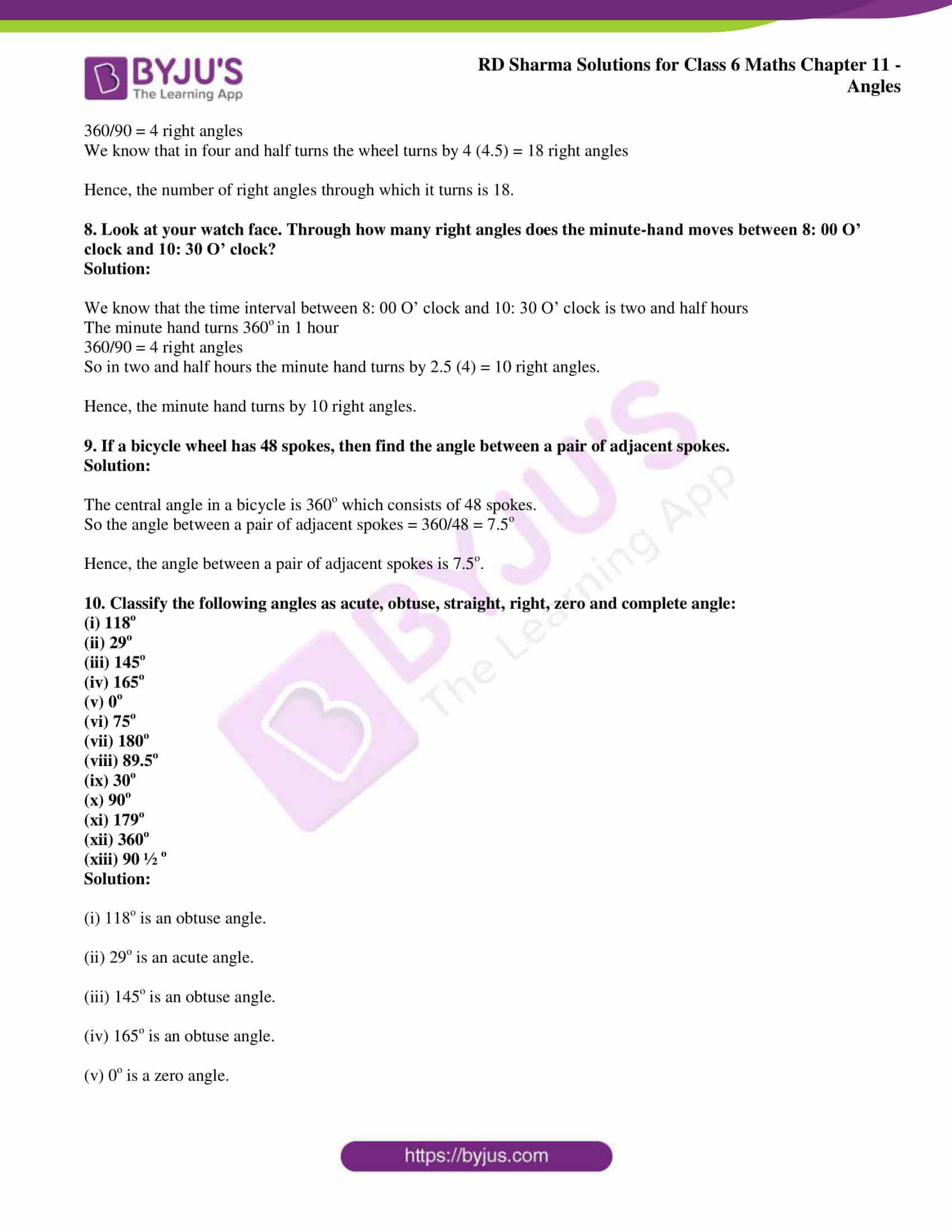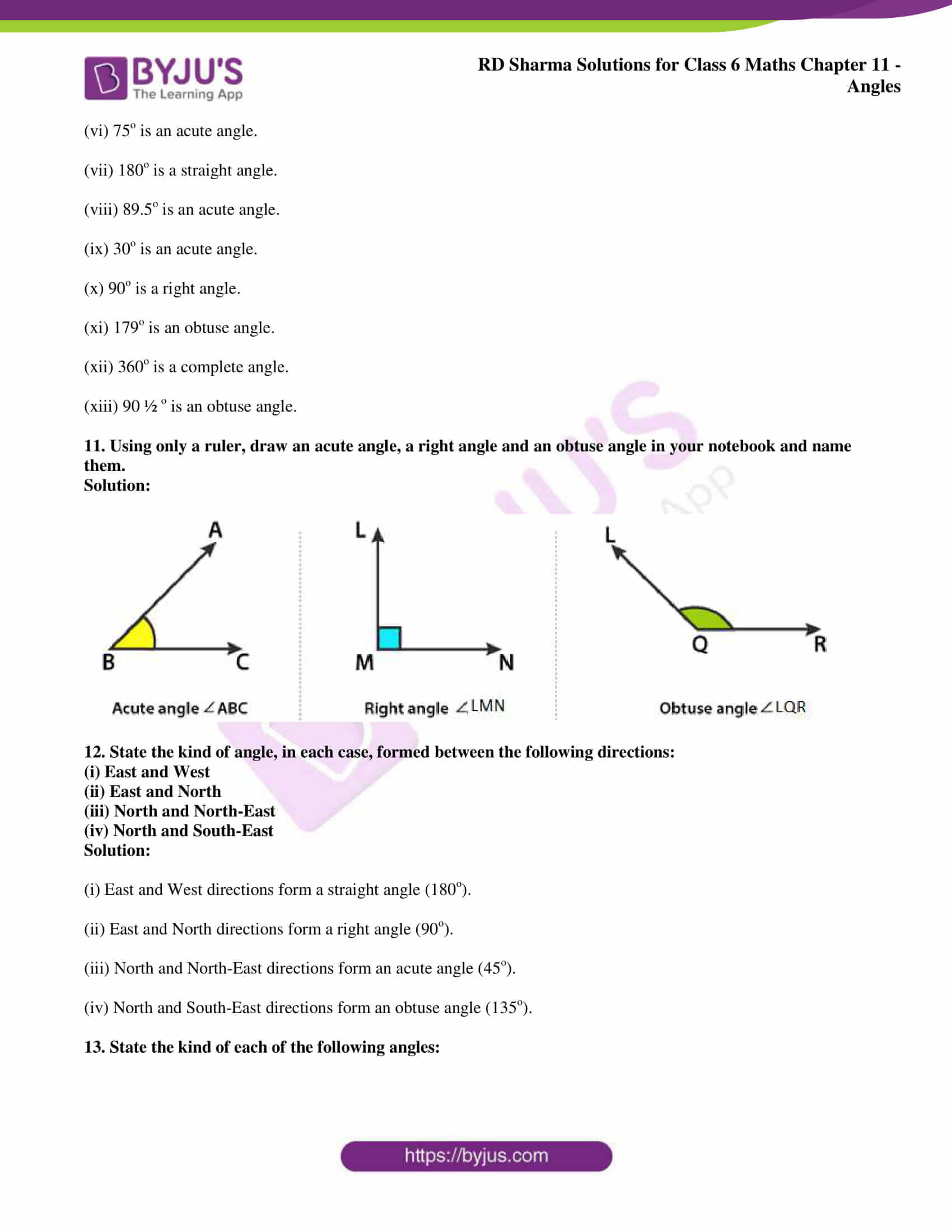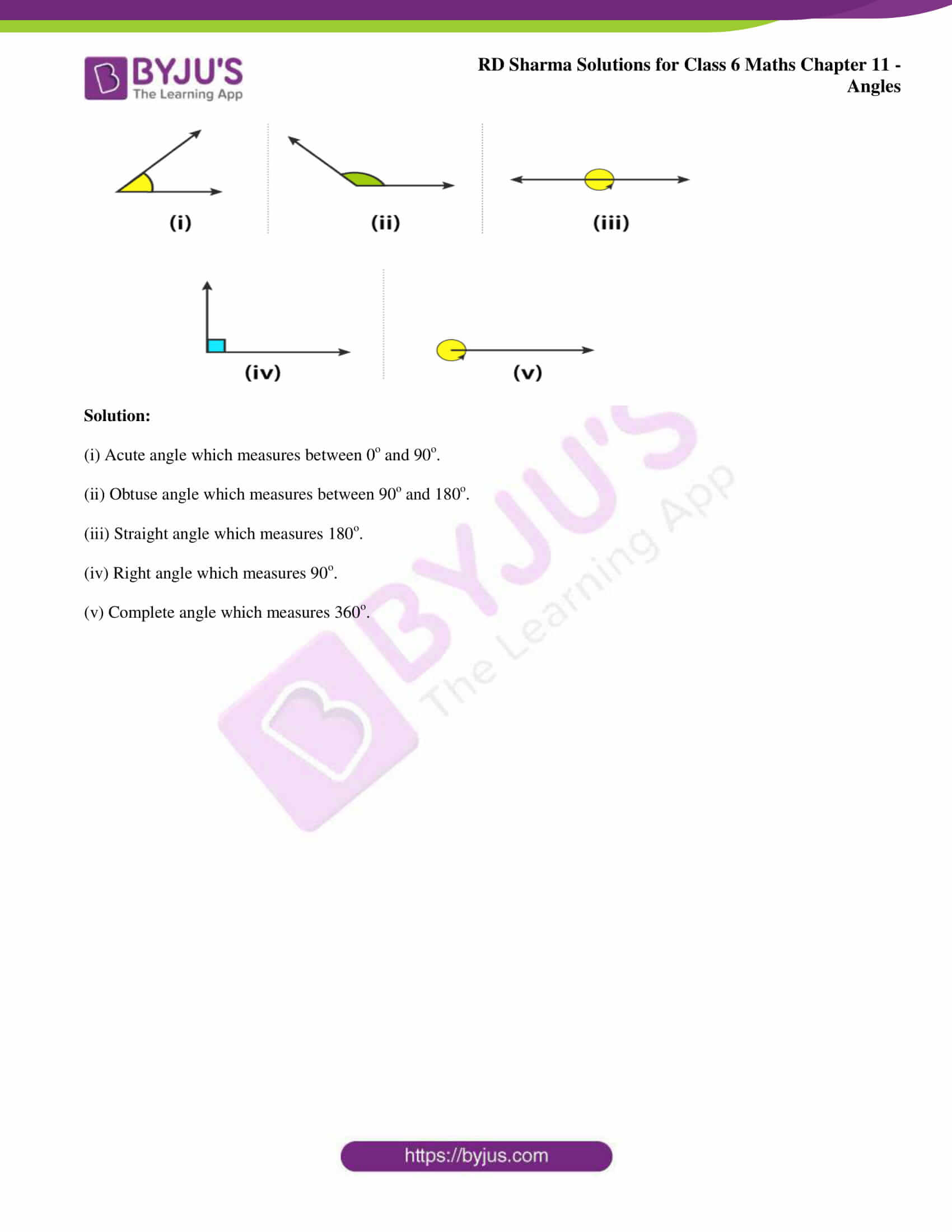### Access answers to Maths RD Sharma Solutions for Class 6 Chapter 11: Angles Exercise 11.2

1. Give two examples each of right, acute and obtuse angles from your environment.

Solution:

The two examples of right angle are:

Two adjacent walls of a room and adjacent edges of a book

The two examples of acute angle are:

Two adjacent sides of the letter Z and two adjacent fingers of our hand.

The two examples of obtuse angles are:

Two sloping sides of a roof and two adjacent blades of a fan.

2. An angle is formed by two adjacent fingers. What kind of angle will it appear?

Solution:

The angle formed by two adjacent fingers will appear as acute angle.

3. Shikha is rowing a boat due north-east. In which direction will she be rowing if she turns it through:

(i) a straight angle

(ii) a complete angle.

Solution:

(i) If she turns through a straight angle (180o) she will be rowing in the South-West direction.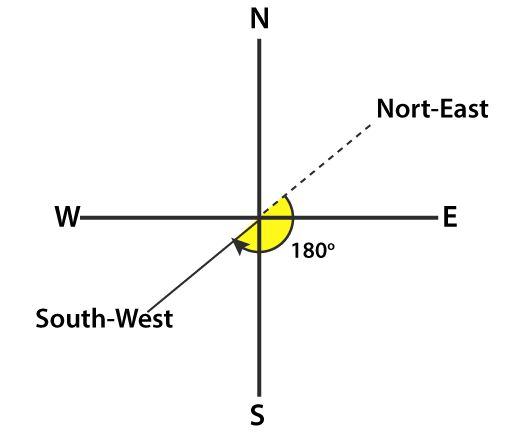(ii) If she turns through a complete angle (360o) she will be rowing in North-East direction.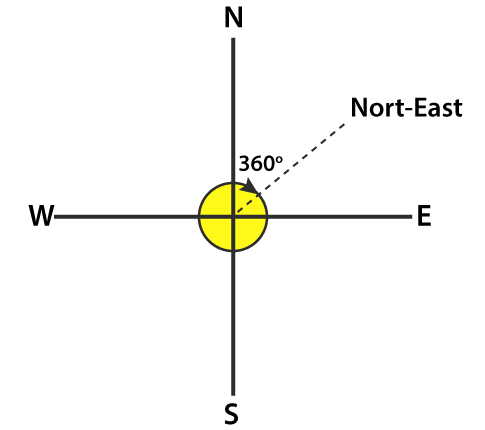4. What is the measure of the angle in degrees between:

(i) North and West?

(ii) North and South?

(iii) North and South- East?

Solution: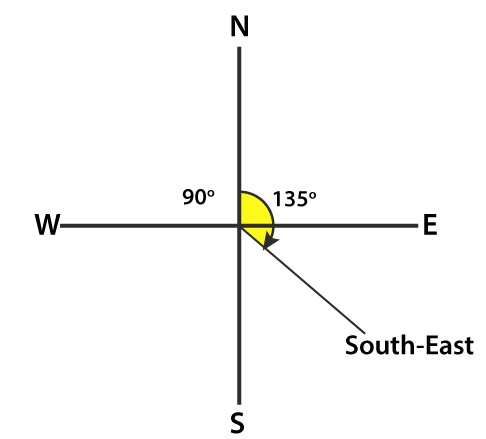The measure of the angle in degrees between:

(i) North and West is 90o.

(ii) North and South is 180o.

(iii) North and South-East is 135o.

5. A ship sailing in river Jhelam moves towards east. If it changes to north, through what angle does it turn?

Solution:

It the ship moves from east to north direction, the angle it turns is 90o.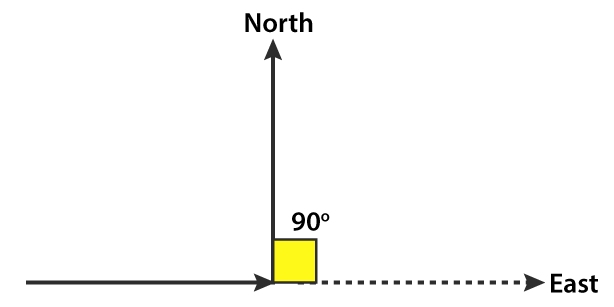6. You are standing in a class-room facing north. In what direction are you facing after making a quarter turn?

Solution:

By making a quarter turn (90o), I will be facing towards east if I turn to my right hand and if I turn to my left hand, I will be facing towards west.

7. A bicycle wheel makes four and a half turns. Find the number of right angles through which it turns.

Solution:

We know that the wheel of a bicycle covers 360o in one turn.

It can be written as

360/90 = 4 right angles

We know that in four and half turns the wheel turns by 4 (4.5) = 18 right angles

Hence, the number of right angles through which it turns is 18.

8. Look at your watch face. Through how many right angles does the minute-hand moves between 8: 00 O’ clock and 10: 30 O’ clock?

Solution:

We know that the time interval between 8: 00 O’ clock and 10: 30 O’ clock is two and half hours

The minute hand turns 360o in 1 hour

360/90 = 4 right angles

So in two and half hours the minute hand turns by 2.5 (4) = 10 right angles.

Hence, the minute hand turns by 10 right angles.

9. If a bicycle wheel has 48 spokes, then find the angle between a pair of adjacent spokes.

Solution:

The central angle in a bicycle is 360o which consists of 48 spokes.

So the angle between a pair of adjacent spokes = 360/48 = 7.5o

Hence, the angle between a pair of adjacent spokes is 7.5o.

10. Classify the following angles as acute, obtuse, straight, right, zero and complete angle:

(i) 118o

(ii) 29o

(iii) 145o

(iv) 165o

(v) 0o

(vi) 75o

(vii) 180o

(viii) 89.5o

(ix) 30o

(x) 90o

(xi) 179o

(xii) 360o

(xiii) 90 ½ o

Solution:

(i) 118o is an obtuse angle.

(ii) 29o is an acute angle.

(iii) 145o is an obtuse angle.

(iv) 165o is an obtuse angle.

(v) 0o is a zero angle.

(vi) 75o is an acute angle.

(vii) 180o is a straight angle.

(viii) 89.5o is an acute angle.

(ix) 30o is an acute angle.

(x) 90o is a right angle.

(xi) 179o is an obtuse angle.

(xii) 360o is a complete angle.

(xiii) 90 ½ o is an obtuse angle.

11. Using only a ruler, draw an acute angle, a right angle and an obtuse angle in your notebook and name them.

Solution: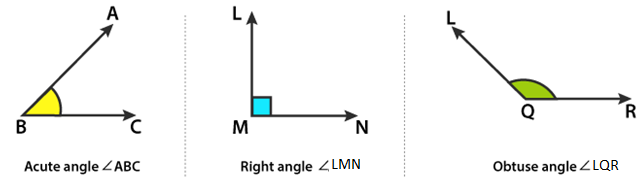12. State the kind of angle, in each case, formed between the following directions:

(i) East and West

(ii) East and North

(iii) North and North-East

(iv) North and South-East

Solution:

(i) East and West directions form a straight angle (180o).

(ii) East and North directions form a right angle (90o).

(iii) North and North-East directions form an acute angle (45o).

(iv) North and South-East directions form an obtuse angle (135o).

13. State the kind of each of the following angles: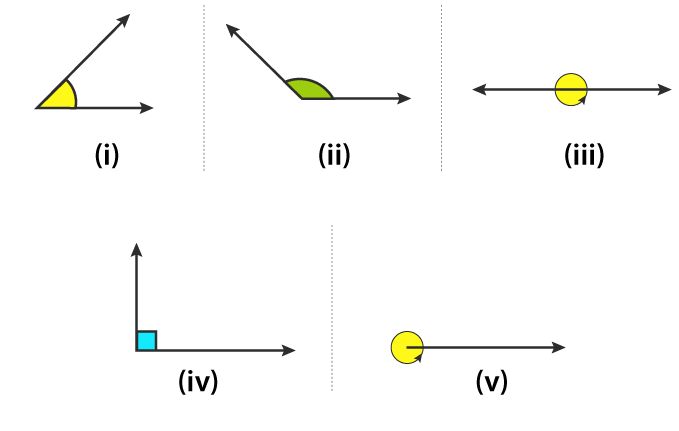Solution:

(i) Acute angle which measures between 0o and 90o.

(ii) Obtuse angle which measures between 90o and 180o.

(iii) Straight angle which measures 180o.

(iv) Right angle which measures 90o.

(v) Complete angle which measures 360o.